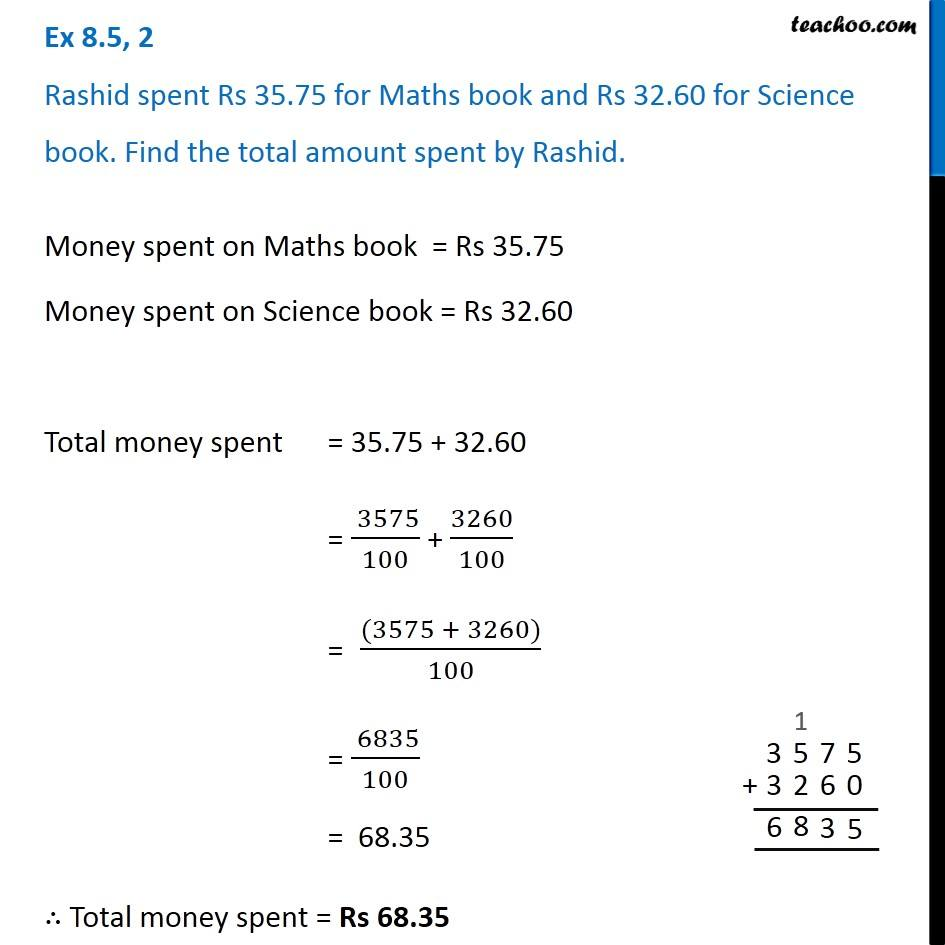Addition of decimal numbers - Statement questions

Chapter 8 Class 6 Decimals
Concept wiseLearn in your speed, with individual attention - Teachoo Maths 1-on-1 Class

### Transcript

Ex 8.3, 2 Rashid spent Rs 35.75 for Maths book and Rs 32.60 for Science book. Find the total amount spent by Rashid.Money spent on Maths book = Rs 35.75 Money spent on Science book = Rs 32.60 Total money spent = 35.75 + 32.60 = ( 3575)/100 + 3260/100 = ((3575 + 3260))/100 = ( 6835)/100 = 68.35 ∴ Total money spent = Rs 68.35